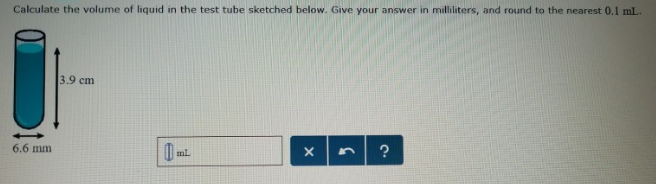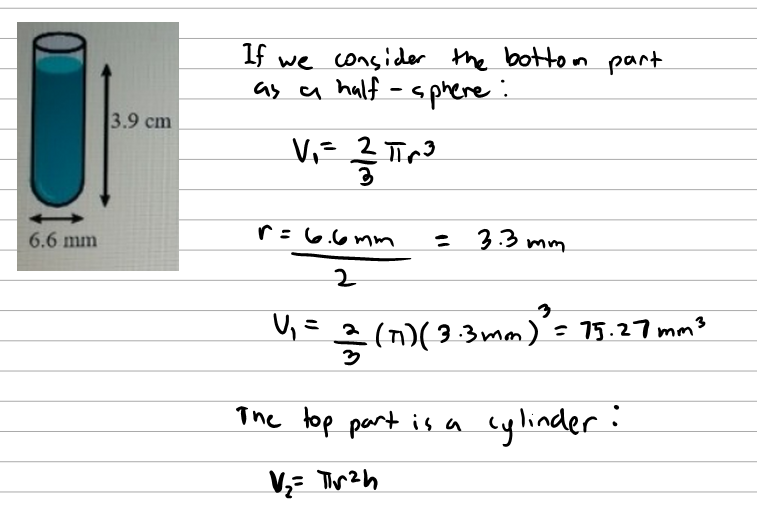Chemistry Practice Problems Metric Prefixes Practice Problems Solution: Calculate the volume of liquid in the test tube sk...

🤓 Based on our data, we think this question is relevant for Professor Bowers' class at KENT.

# Solution: Calculate the volume of liquid in the test tube sketched below. Give your answer in milliliters, and round to the nearest 0.1 mL.

###### Problem

Calculate the volume of liquid in the test tube sketched below. Give your answer in milliliters, and round to the nearest 0.1 mL.###### SolutionView Complete Written Solution

Metric Prefixes

Metric Prefixes

#### Q. A newborn is 18 in. in length and weighs 8 lb 13 oz. Describe the baby in metric units. Be sure to answer all parts.Enter your answer in scientific no...

Solved • Fri Sep 07 2018 12:01:07 GMT-0400 (EDT)

Metric Prefixes

#### Q. The radius of a lead atom is 175 pm. How many lead atoms would have to be laid side by side to span a distance of 2.37 mm?

Solved • Thu Sep 06 2018 12:57:05 GMT-0400 (EDT)

Metric Prefixes

#### Q. Calculate the volume of liquid in the round flask sketched below. Give your answer in milliliters, and round to the nearest 0.1 mL.

Solved • Wed Sep 05 2018 15:16:43 GMT-0400 (EDT)

Metric Prefixes

#### Q. Which is the correct proportionality factor relating pg (picogram) and g? (a) 109 pg/1 g(b) 1 pg/1012 g (c) 1015 pg = 1 mg (d) 1012 pg/1 g

Solved • Thu Aug 23 2018 13:16:26 GMT-0400 (EDT)